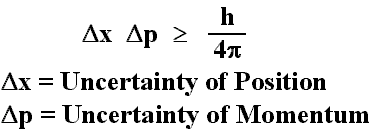Search
•Oshin Mittal

# A Brief History of Time | Chapter 4: The Uncertainty Principle | Summary

Till now we saw about different properties of light, but the question arises what is light? Can we predict the future? In 1900 Max Planck suggested that any electromagnetic wave was emitted in the form of quanta, which were kind of energy packet. Thus, greater the frequency is, more the number of quanta hitting per unit time and hence the rate at which a body will lose its energy was predominately finite. In 1926 in an attempt to predict future position, Werner Heisenberg experienced that in order to measure distance or position, one will have to use the light of shorter wavelength and hence high energy single quanta. This high energy quanta will disturb the particle’s velocity by a considerable amount and converse is the case when one tries to find the velocity. Thus, concluding that one can never measure both the position and velocity accurately at the same time. The accuracy in measurement in either quantity comes at the cost of variation of the other. Heisenberg formulated that the product of discrepancy in the position and the variation in the velocity of an object is always less than a constant value named Planck’s constant. This came to be known as Heisenberg’s Uncertainty Principle.In 1920s Heisenberg’s, Erwin Schrodinger and Paul Dirac reformulated mechanics to Quantum Mechanics, a theory based on the uncertainty principle. This theory didn’t depend on well-defined velocities and position but on the quantum state which was the combination of the two. Quantum mechanics introduced an unavoidable element of unpredictability or randomness into science. In general, it does not predict a single definite result for observation but a number of different possible outcomes and their probabilities of occurrence. This theorem brings into the picture the wave-particle duality. Quantum mechanics also resolved the difficulties that arose in Bohr’s atomic model.

Today quantum mechanics governs the principle of working of all aspects of science and technology ranging from integrated circuits to modern chemistry and biology. The only areas where quantum mechanics has still not been able to incorporate are gravity and the large-scale structure of the universe.

[ A chapter’s summary from the great book A Brief History of Time -By Stephen Hawkins].

Summary Ch4 The Uncertainty Principle(MP
.